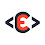# Create The Tetris Game Using JavaScript

In general, to create a JavaScript game, you'll need two things, the first is the HTML5 canvas, and the second is JavaScript.

At anytime you can go below, and

First things first, this is what you need to know about the Tetris game.

The game board: the game is played on a board, that has 20 rows and 10 columns. in programming languages that's a 2D array.

Tetrominoes: are the pieces we play with, there are 7 different pieces, Z, T, L, O, I, J, and S. the pieces can move to the right, to the left, to the bottom, and rotate. and when a Tetromino reaches the bottom we lock it to the board, and we ask for a new one.

Walls: we have two walls, the right and the left wall.

Square: the board and the Tetrominoes, are both made of squares, a square by default is 20px by 20px rectangle.

Create the PIECES (or tetrominoes).

let's take  the Z piece as an example. this how it looks like.
The idea is, for a VACANT(empty) square we give 0, and for an OCCUPIED square we give 1.
Now this is just one pattern out of four, the code for this pattern is this.
Remember the player should be able to rotate a piece, the easiest way to do that with code, is to put all the patterns of a Tetromino inside an array.
So we need to create an array for each Tetromino, and here is the example for the Z piece.

Now you should be able to figure out the code for all the other pieces. but it's not really necessary to type in the code all over again, since I already did that. actually you don't have to type in any code for now, all you have to do for now is to understand the logic behind the game, now that you understand how we create the pieces using code, let's move and see how to create our board.
Create the GAME BOARD.

The board is a 2D array, means an array inside another array, which means we will need two for loops, the first for loop, will create the rows, and the second will create the columns.

a 20px/20px SQUARE is our UNIT.

Since the board and the pieces are both made of squares, we will use a constant called SQ (for square) = 20, as a unit.

So using code we will say : const SQ = 20;

How to draw a SQUARE to the CANVAS? We need First to go and create the canvas element, inside our index.HTML file :

Then before the closing body tag "</body>", we add our JavaScript code.

Now we need to write our code, inside tetris.js file, the first thing that we will do, is to select our canvas, and getContext('2d') of our canvas :

getContext('2d') method, has properties and methods that allow us to draw and DO different things on the canvas.

Let's now talk about how to draw a square. a square is a rectangle for that,we will use a method, which is; ctx.fillRect(X,Y,W,H); X and Y, are the square position, and W is the width of the square, and H is the height of the square.

Before that, we need to define the color with, we want to draw our square, so we need to say; ctx.fillStyle = "red"; the square must now has a red color.

So if you say;

We will get a square at the top left corner, with a red color, and width and height both equal to 20 pixels.

To add a stroke for that square is so simple.

Now we should see our square with black stroke.

To make this code reusable, we just need to put it inside a function called drawSquare, this function will get as arguments, the X and Y position, and the color.

Now to draw the square above, all we need to say is, drawSquare(0, 0, "red"), and we will get the same result.

I think you're now ready to watch my tutorial about how to create the Tetris game using JavaScript.
First download the starter template from gitHub, so you can follow the tutorial step by step :

The Game link : Code Explained Repo

Other Tutorials for games created using JavaScript :

Create the Snake Game Using Javascript

Create the Flappy Bird Game Using Javascript

1.Awesome Tutorials..

2.Thanks you :)

3.Excellent tutorials! I love the way you explain the logic before getting into hands on coding

1.4.This comment has been removed by the author.

5.Great tutorial ..
However, there are many ways to code Tetris and we should get the same result , but I think it may be much easier to use CSS grid instead of canvas, because the grid is exactly what you need for building squire shapes controlled by x, y start points . Also the second benefit for using CSS grid is the easy way of building responsive layout using hv, wv, vmin, etc

thank you again

6.Thanks for this buddy